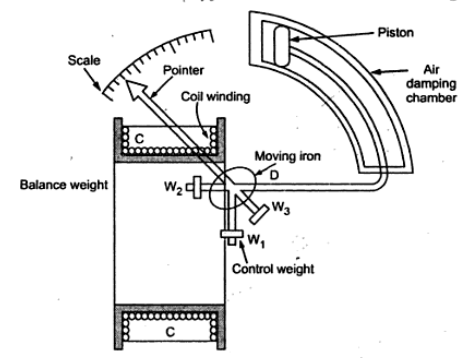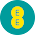### Moving Iron Instruments

The moving iron instruments are classified as:
i) Moving iron attraction type instruments and
ii) Moving iron repulsion type instruments
1.1 Moving iron attraction type instruments
The basic working principle of these instruments is very simple that a soft iron piece if brought near the magnet gets attracted by the magnet.
The construction of the attraction type instrument is shown in the Fig.1.Fig. 1 Moving iron attraction type instruments
It consists of a fixed coil C and moving iron piece D. The coil is flat and has a narrow slot like opening. The moving iron is a flat disc which eccentrically mounted. on the spindle. The spindle is supported between the jewel bearings. The spindle caries a pointer which moves over a graduated scale. The number of turns of the fixed coil are dependent on the range of the instrument. For passing current through the coil only few turns are required.
The controlling torque is provided by the springs but gravity control may also ne used for vertically mounted panel type instruments.
The damping torque is provided by the air friction. A light aluminium piston is attached to the moving system. It moves in a fixed chamber. The chamber is closed at one end. It can also provided with the help of van attached to the moving system.
The operating magnetic field in moving iron instruments is very weak. Hence eddy current damping is not used since it require a permanent magnet which would  affect or distort the operating field.
1.2 Moving Iron Repulsion Type Instrument
These instruments have two vanes inside the coil, the one is fixed and other is movable. When the current flows in the coil, both the vanes are magnetised with like polarities induced on the same side. Hence due to repulsion of like polarities, there is a force of repulsion between the two vanes causing the movement of the moving van. The repulsion type instruments are the most commonly used instruments.
The two different designs of repulsion type instruments are:
ii) Co-axial vane type
1.2.1 Radial Van Repulsion Type Instrument
The Fig. 2 shows the radial vane repulsion type instrument. Out of the other moving iron mechanism, this is the most sensitive and has most linear scale.Fig. 2 Radial Van Repulsion Type Instrument

The two vanes are radial strips of iron. The fixed vane is attached to the coil. The movable vane is attached to the spindle and suspended in the induction field of the coil. The needle of the instrument is attached to this vane.
Eventhough the current through the coil is alternating, there is always repulsion between the like poles of the fixed and the movable vane. Hence the deflection of the pointer is always in the same direction. The deflection is effectively proportional to the actual current and hence the scale is calibrated directly to rad amperes or volts. The calibration is accurate only for the frequency for which it is designed because the impedance is different for different frequencies.
1.2.2 Concentric Vane Repulsion Type Instrument
Fig. 3 shows the concentric vane repulsion type instrument. The instrument has two concentric vanes. One is attached to the coil frame rigidly while the other can rotate coaxially inside the stationary vane.Fig. 3 Concentric Vane Repulsion Type Instrument

Both the vanes are magnetised to the same polarity due to the current in the coil. Thus the movable vane rotates under the repulsive force. As the movable vane is attached to the pivoted shaft, the repulsion results in a rotation of the shaft. The pointer deflection is proportional to the current in the coil. The concentric vane type instrument is moderately sensitive and the deflection is proportional to the square of the current through coil. Thus the instrument said to have square low response. Thus the scale of the instrument is non-uniform in nature. Thus whatever may be the direction of the current in the coil, the deflection in the moving iron instruments is in the same direction. Hence moving iron instruments can be used for both a.c. and d.c. measurements. Due to square low response, the scale of the moving iron instrument is non-uniform.
1.3 Torque Equation of moving iron instruments
Consider a small increment in current supplied to the coil of the instrument. due to this current let dθ be the deflection under the deflecting torque Td. Due to such deflection, some mechanical work will be done.
...          Mechanical Work = Td  dθ
There will be a change in the energy stored i the magnetic field due to the change in inductance. This is because the vane tries to occupy the position of minimum reluctance. The inductance is inversely proportional to the reluctance of  the magnetic circuit of coil.
Let   I = initial current
L = instrument inductance
θ = deflection
dI = increase in current
dθ = change in deflection
dL = change in inductance
In order to effect an increment dL in the current, there must be an increase in the applied voltage given by,

The electrical energy supplied is given by,

Hence the change in stored energy is given by,

The energy supplied in nothing but increase in stored energy plus the energy required for mechanical work done.

While the controlling torque is given by,

Thus the deflection is proportional to the square of the current through the coil. And the instrument gives square law response.
The various advantages of moving iron instruments are,
1) The instruments can be used for both a.c. and d.c. measurements.
2)As the torque to weight ratio is high, errors due to the friction are very less.
3) A single type of moving element can cover the wide range hence these instruments are cheaper than other types of if instruments.
4) There are no current carrying parts in the moving system hence these meters are extremely rugged and reliable.
5) These are capable of giving good accuracy. Modern moving iron instruments have a d.c. error of 2% or less.
6) These can withstand large loads and are not damaged even under sever overload conditions.
7) The range of instruments can be extended.
The various disadvantages of moving iron instruments are,
1) The scale of moving iron instruments is not uniform and is cramped at the lower end. Hence accurate readings are not possible at this end.
2) There are serious errors due to hysteresis, frequency changes and stray magnetic fields.
3) The increase in temperature increases the resistance of coil, decreases stiffness of the springs, decreases the permeability and hence affect the reading severely.
4) Due to the non linearity of B-H curve, the deflecting torque is not exactly proportional to the square of the current.
5) There is a difference between a.c. and d.c. calibration on account of the effect of inductance of the meter. Hence these meters must always be calibrated at the frequency at which they are to be used. The usual commercial moving iron instrument may be used within its specified accuracy from 25 to 125 HZ frequency range.
6) Power consumption is on higher side.
1.6 Errors in moving iron instruments
The various errors in the moving iron instruments are,
1) Hysteresis error: Due to hysteresis effect, the flux density for the same current while ascending and descending values is different. While descending, the flux density is higher and while ascending it is lesser. So meter reads higher for descending values of current or voltage. So remedy for this is to use smaller iron parts which can demagnetise quickly or to work with lower flux densities.
2) Temperature error : The temperature error arises due to the effect of temperature on the temperature coefficient of the spring. This error is of the order of 0.02 % per oC change in temperature. Errors can cause due to self heating of the coil and due to which change in resistance of the coil. So coil and series resistance must have low temperature coefficient. Hence mangnin is generally used for the series resistance.
3) Stray magnetic Field Error : The operating magnetic field in case of moving iron instruments is very low. Hence effect of external i.e. stray magnetic field can cause  error. This effect depends on the direction of the stray magnetic field with respect to the operating field of the instrument.
4) Frequency Error : These are related to a.c. operation of the instrument. The change in frequency affects the reactance of the working coil and also affects the magnitude of the eddy currents. This cause error in the instrument.
5) Eddy Current Error : When instrument is used for a.c. measurements the eddy currents are produced in the iron parts of the instrument. The eddy current affects the instrument current causing the change in the deflection torque. This produce the error in the meter reading. As eddy current are frequency dependent, frequency changes cause eddy current error.

#### 1 comment:

1.